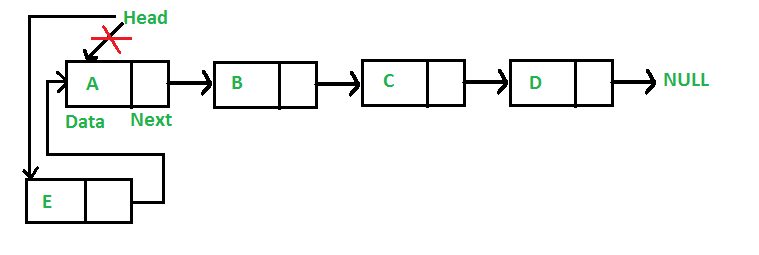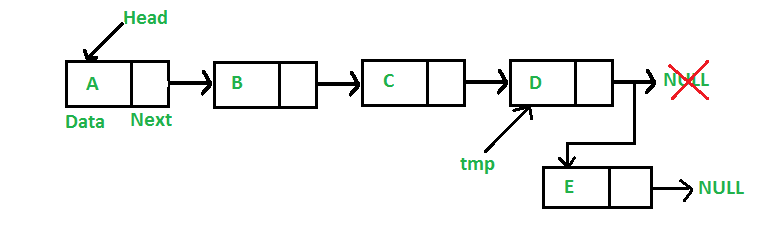Open in App
Not now

# Java Program For Inserting A Node In A Linked List

• Difficulty Level : Easy
• Last Updated : 01 Sep, 2022

We have introduced Linked Lists in the previous post. We also created a simple linked list with 3 nodes and discussed linked list traversal.
All programs discussed in this post consider the following representations of the linked list.

## Java

 `// Linked List Class` `class` `LinkedList` `{` `    ``// Head of list` `    ``Node head;  `   `    ``// Node Class ` `    ``class` `Node` `    ``{` `        ``int` `data;` `        ``Node next;` `         `  `        ``// Constructor to create` `        ``// a new node` `        ``Node(``int` `d) ` `        ``{` `            ``data = d; ` `            ``next = ``null``; ` `        ``}` `    ``}` `}`

In this post, methods to insert a new node in linked list are discussed. A node can be added in three ways
1) At the front of the linked list
2) After a given node.
3) At the end of the linked list.

Add a node at the front: (4 steps process)Following are the 4 steps to add a node at the front.

## Java

 `/* This function is in LinkedList class. Inserts a` `   ``new Node at front of the list. This method is ` `   ``defined inside LinkedList class shown above */` `public` `void` `push(``int` `new_data)` `{` `    ``/* 1 & 2: Allocate the Node &` `              ``Put in the data*/` `    ``Node new_node = ``new` `Node(new_data);`   `    ``// 3. Make next of new Node as head ` `    ``new_node.next = head;`   `    ``// 4. Move the head to point to ` `    ``// new Node ` `    ``head = new_node;` `}`

Time complexity of push() is O(1) as it does a constant amount of work.
Add a node after a given node: (5 steps process)
We are given a pointer to a node, and the new node is inserted after the given node.

## Java

 `/* This function is in LinkedList class. ` `   ``Inserts a new node after the given ` `   ``prev_node. This method is defined ` `   ``inside LinkedList class shown above */` `public` `void` `insertAfter(Node prev_node, ` `                        ``int` `new_data) ` `{ ` `    ``// 1. Check if the given Node is null ` `    ``if` `(prev_node == ``null``) ` `    ``{ ` `        ``System.out.println(` `               ``"The given previous node cannot be null"``); ` `        ``return``; ` `    ``} `   `    ``/* 2. Allocate the Node & ` `       ``3. Put in the data*/` `    ``Node new_node = ``new` `Node(new_data); `   `    ``// 4. Make next of new Node as next` `    ``// of prev_node ` `    ``new_node.next = prev_node.next; `   `    ``// 5. make next of prev_node as new_node ` `    ``prev_node.next = new_node; ` `}`

Time complexity of insertAfter() is O(1) as it does a constant amount of work.

Add a node at the end: (6 steps process)
The new node is always added after the last node of the given Linked List. For example if the given Linked List is 5->10->15->20->25 and we add an item 30 at the end, then the Linked List becomes 5->10->15->20->25->30.
Since a Linked List is typically represented by the head of it, we have to traverse the list till the end and then change the next to last node to a new node.Following are the 6 steps to add node at the end.

## Java

 `/* Appends a new node at the end.  This method is ` `   ``defined inside LinkedList class shown above */` `public` `void` `append(``int` `new_data)` `{` `    ``/* 1. Allocate the Node &` `       ``2. Put in the data` `       ``3. Set next as null */` `    ``Node new_node = ``new` `Node(new_data);`   `    ``/* 4. If the Linked List is empty, then ` `          ``make the new node as head */` `    ``if` `(head == ``null``)` `    ``{` `        ``head = ``new` `Node(new_data);` `        ``return``;` `    ``}`   `    ``/* 4. This new node is going to be the ` `          ``last node, so make next of it ` `          ``as null */` `    ``new_node.next = ``null``;`   `    ``// 5. Else traverse till the last node ` `    ``Node last = head; ` `    ``while` `(last.next != ``null``)` `        ``last = last.next;`   `    ``// 6. Change the next of last node ` `    ``last.next = new_node;` `    ``return``;` `}`

Time complexity of append is O(n) where n is the number of nodes in the linked list. Since there is a loop from head to end, the function does O(n) work.
This method can also be optimized to work in O(1) by keeping an extra pointer to the tail of the linked list/

Following is a complete program that uses all of the above methods to create a linked list.

## Java

 `// A complete working Java program to ` `// demonstrate all insertion methods` `// on linked list` `class` `LinkedList` `{` `    ``// head of list` `    ``Node head;  `   `    ``// Linked list Node` `    ``class` `Node` `    ``{` `        ``int` `data;` `        ``Node next;` `        ``Node(``int` `d) ` `        ``{` `            ``data = d; ` `            ``next = ``null``; ` `        ``}` `    ``}`   `    ``// Inserts a new Node at front ` `    ``// of the list. ` `    ``public` `void` `push(``int` `new_data)` `    ``{` `        ``/* 1 & 2: Allocate the Node &` `                  ``Put in the data*/` `        ``Node new_node = ``new` `Node(new_data);`   `        ``// 3. Make next of new Node as head ` `        ``new_node.next = head;`   `        ``// 4. Move the head to point to` `        ``// new Node ` `        ``head = new_node;` `    ``}`   `    ``// Inserts a new node after the ` `    ``// given prev_node. ` `    ``public` `void` `insertAfter(Node prev_node, ` `                            ``int` `new_data)` `    ``{` `        ``// 1. Check if the given Node is null ` `        ``if` `(prev_node == ``null``)` `        ``{` `            ``System.out.println(` `                   ``"The given previous node cannot be null"``);` `            ``return``;` `        ``}`   `        ``/* 2 & 3: Allocate the Node &` `                  ``Put in the data*/` `        ``Node new_node = ``new` `Node(new_data);`   `        ``// 4. Make next of new Node as next ` `        ``// of prev_node ` `        ``new_node.next = prev_node.next;`   `        ``// 5. make next of prev_node as ` `        ``// new_node ` `        ``prev_node.next = new_node;` `    ``}` `   `  `    ``/* Appends a new node at the end. ` `       ``This method is defined inside ` `       ``LinkedList class shown above */` `    ``public` `void` `append(``int` `new_data)` `    ``{` `        ``/* 1. Allocate the Node &` `           ``2. Put in the data` `           ``3. Set next as null */` `        ``Node new_node = ``new` `Node(new_data);`   `        ``/* 4. If the Linked List is empty, ` `              ``then make the new node as head */` `        ``if` `(head == ``null``)` `        ``{` `            ``head = ``new` `Node(new_data);` `            ``return``;` `        ``}`   `        ``/* 4. This new node is going to be ` `              ``the last node, so make next ` `              ``of it as null */` `        ``new_node.next = ``null``;`   `        ``// 5. Else traverse till the last node ` `        ``Node last = head; ` `        ``while` `(last.next != ``null``)` `            ``last = last.next;`   `        ``// 6. Change the next of last node ` `        ``last.next = new_node;` `        ``return``;` `    ``}`   `    ``/* This function prints contents of ` `       ``linked list starting from the ` `       ``given node */` `    ``public` `void` `printList()` `    ``{` `        ``Node tnode = head;` `        ``while` `(tnode != ``null``)` `        ``{` `            ``System.out.print(tnode.data + ``" "``);` `            ``tnode = tnode.next;` `        ``}` `    ``}`   `    ``// Driver code` `    ``public` `static` `void` `main(String[] args)` `    ``{` `        ``// Start with the empty list ` `        ``LinkedList llist = ``new` `LinkedList();`   `        ``// Insert 6. So linked list ` `        ``// becomes 6->NUllist` `        ``llist.append(``6``);`   `        ``// Insert 7 at the beginning. ` `        ``// So linked list becomes ` `        ``// 7->6->NUllist` `        ``llist.push(``7``);`   `        ``// Insert 1 at the beginning. ` `        ``// So linked list becomes` `        ``// 1->7->6->NUllist` `        ``llist.push(``1``);`   `        ``// Insert 4 at the end. ` `        ``// So linked list becomes` `        ``// 1->7->6->4->NUllist` `        ``llist.append(``4``);`   `        ``// Insert 8, after 7. So linked ` `        ``// list becomes` `        ``// 1->7->8->6->4->NUllist` `        ``llist.insertAfter(llist.head.next, ``8``);`   `        ``System.out.println(` `               ``"Created Linked list is: "``);` `        ``llist.printList();` `    ``}` `}` `// This code is contributed by Rajat Mishra`

Output:

` Created Linked list is:  1  7  8  6  4`

Time complexity: O(N) where N is size of given linked list

Auxiliary space: O(1) constant space is being used

Please refer complete article on Linked List | Set 2 (Inserting a node) for more details!

My Personal Notes arrow_drop_up
Related Articles